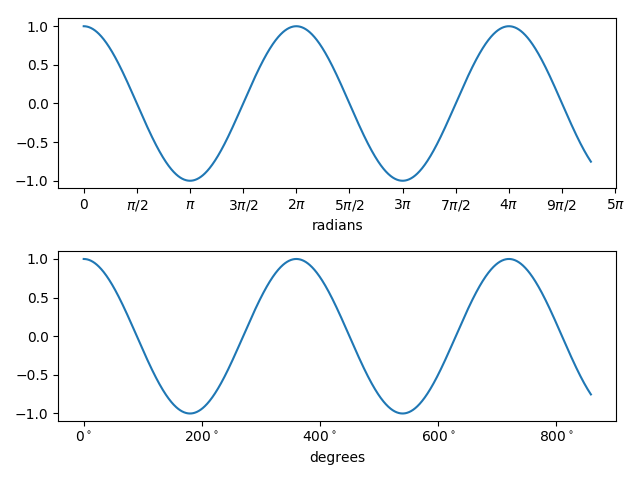### Related Topics

Plot with radians from the basic_units mockup example package.

This example shows how the unit class can determine the tick locating, formatting and axis labeling.

This example requires basic_units.pyimport matplotlib.pyplot as plt
import numpy as np

from basic_units import radians, degrees, cos

x = [val*radians for val in np.arange(0, 15, 0.01)]

fig, axs = plt.subplots(2)